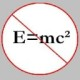You are currently browsing the daily archive for March 8, 2008.

1. Notions of Set Theory

Artin introduces a concept that is referred to as the canonical factoring of a map (function). The basic idea is that any function$f$ can be factored into three functions$f_1, f_2$ and$f_3$ in a somewhat unique way:$f = f_3 f_2 f_1$, where$f_1$ is onto,$f_2$ is a bijection, and$f_3$ is an injection. The construction of these three functions is done in a canonical, or natural, way that doesn’t require the use of objects outside the domain and/or range of$f$.

Let$S$ be some non-empty set. If$f$ is a function from$S$ into a set$T$, then we write$f: S \to T$.

Suppose$f: S \to T$ and$g: T \to U$. Then, we can form a composite function$g \circ f: S \to U$ defined by$(g \circ f)(s) = g(f(s))$ for all$s \in S$. The associative law holds trivially for composition of functions.

Further, if$S_0 \subset S$, then the set of all the images of elements of$S_0$, denoted by$f(S_0)$, is called the image of$S_0$. In general,$f(S) \subset T$. We call the function$f$ onto whenever$f(S) = T$.

Now, let us partition the set$S$ into equivalence classes such that$s_1, s_2 \in S$ are in the same equivalence class iff$f(s_1) = f(s_2)$. This partition is called the quotient set and is denoted by$S_f$.

To illustrate, suppose$S = \{ 1, 2, 3, 4\}$ and$T = \{ a, b, c, d\}$. Also, let$f: S \to T$ such that$f(1) = a, f(2) = b, f(3) = b$ and$f(4) = c$. Then, the quotient set,$S_f = \{ \{ 1\}, \{ 2, 3\}, \{ 4\}\}$.

We construct now a function$f_1: S \to S_f$ that maps each$s \in S$ to its equivalence class. It can be verified that$f_1$ is onto. So, taking the above example, we have$f_1(1) = \{ 1 \}$,$f_1(2) = \{ 2, 3\}$,$f_1(3) = \{ 2, 3\}$ and$f_1(4) = \{4\}$.

Next, we construct a function$f_2: S_f \to f(S)$ where each element (which is an equivalence class) of$S_f$ is mapped to a$t \in T$ where each$t$ is the image of the members of the equivalence class. Recall that$s_1, s_2 \in S$ are in the same equivalence class iff$f(s_1) = f(s_2)$. Therefore,$f_2$ is one-to-one and onto. Continuing with our above example, we have$f_2(\{ 1\}) = a$,$f_2(\{ 2, 3\}) = b$ and$f_2(\{ 4\}) = c$.

And, finally, we construct a trivial function$f_3: f(S) \to T$ where$f_3(t) = t$ for each$t \in f(S)$. Note that$f_3$ is not an identity because it maps a subset,$f(S)$, into a possibly larger set,$T$, i.e.$f_3$ is an identity iff$f$ is onto. In general,$f_3$ is one-to-one and into (i.e. an injection.)

Thus, if$f(s) = t$, we note that$f_3 f_2 f_1 (s) = t$ for every$s \in S$.

And, so,$f = f_3 f_2 f_1$.

Once again,$f_1$ is onto,$f_2$ is a bijection, and$f_3$ is an injection.

It looks like it doesn’t make much sense to factor$f$ the way we did above, but we will explore more of this with respect to group homomorphisms in my next post.

### Blog Stats

• 356,110 hitsJohn Favors on Solution to POW-13: Highly…Wayne J. Mann on Solution to POW-12: A graph co…erneststephen on The 54th Carnival of Math…anhtraisg on p^q + q^p is primeprof dr drd horia or… on My first postprof drd horia orasa… on My first postprof dr mircea orasa… on Inequality with lognotedscholar on Self-referential Paradoxes, In…prof dr mircea orasa… on Inequality with logprof dr mircea orasa… on Inequality with logprof dr mircea orasa… on 2010 in reviewkenji on Basic category theory, Iprof dr mircea orasa… on Solution to POW-10: Another ha…prof drd horia orasa… on Continued fraction for eprof drd horia orasa… on Inequality with log